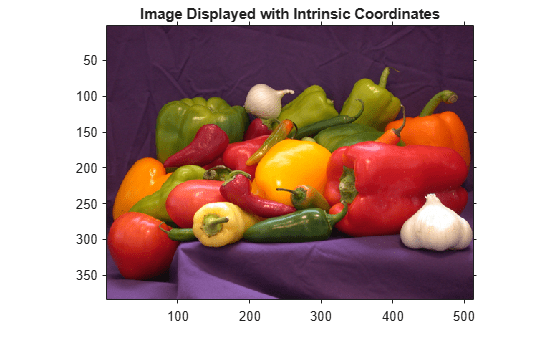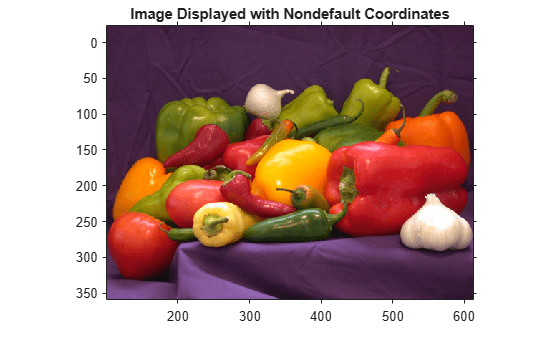# 平移所显示图像的 X 和 Y 坐标范围

`I = imread("peppers.png");`

```figure ax = imshow(I); title("Image Displayed with Intrinsic Coordinates") axis on````xrange = ax.XData`
```xrange = 1×2 1 512 ```
`yrange = ax.YData`
```yrange = 1×2 1 384 ```

```xrangeNew = xrange + 100; yrangeNew = yrange - 25;```

```figure axNew = imshow(I,"XData",xrangeNew,"YData",yrangeNew); title("Image Displayed with Nondefault Coordinates"); axis on````axNew.XData`
```ans = 1×2 101 612 ```
`axNew.YData`
```ans = 1×2 -24 359 ```